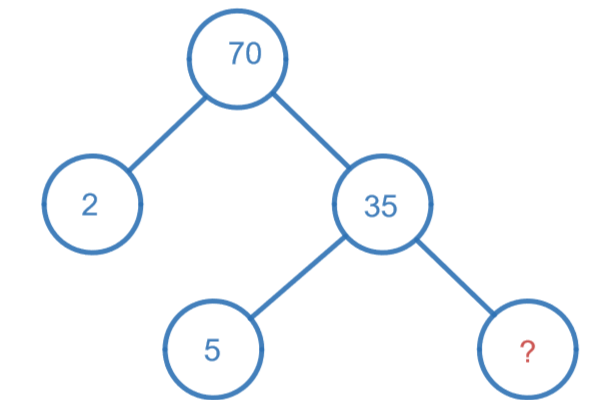# HCF (Highest Common Factor)

## Introduction

HCF (Highest Common Factor) or GCF (Greatest Common Factor) or GCD (Greatest Common Divisor) or GCM (Greatest Common Measure), they all are the same terms. We use them while finding out the number which is the largest common divisor among many divisors of a number.

To see it in more detail how it is calculated, let’s review first some general terms related to HCF.

## Factor

A factor of a number is that number which divides the number exactly.

Example

What are the factors of 40?

There are total eight factors of 40 viz. 1, 2, 4, 5, 8, 10, 20 and 40.

Why?

$$\because$$ these all numbers give remainder zero when 40 is divided by 1, 2, 4, 5, 8, 10, 20 and 40.

How?

$$\frac{40}{1} = 40$$

$$\frac{40}{2} = 20$$

$$\frac{40}{4} = 10$$

$$\frac{40}{5} = 8$$

$$\frac{40}{8} = 5$$

$$\frac{40}{10} = 4$$

$$\frac{40}{20} = 2$$

$$\frac{40}{40} = 1$$

## Common factors

The factors which are common to two or more numbers are called common factors.

Example

Let’s understand it by taking the two numbers as 4 and 10 and further, finding out their common factors.

Step1: find out the factors of 4 and 10 separately.

Factors of 4:

$$\frac{4}{1} = 4$$

$$\frac{4}{2} = 2$$

$$\frac{4}{4} = 1$$

There are total three factors of 4 viz. 1, 2 and 4.

Factors of 10:

$$\frac{10}{1} = 10$$

$$\frac{10}{2} = 5$$

$$\frac{10}{5} = 2$$

$$\frac{10}{10} = 1$$

There are total four factors of 10 viz. 1, 2, 5 and 10.

Step2: Find out the common factors of 4 and 10.

Finally, we can say 1 and 2 are the common factors of 4 and 10, because the factors 1 and 2 do exist for both numbers 4 and 10.

## Highest common factor (HCF)

The number which is greatest among the common factors is called highest common factor (HCF) of two or more numbers.

Example

Let’s learn HCF by an example.

Find the HCF of 12 and 20.

So, what are the steps?

Step 1: Find out the factors for 12.

Step 2: Find out the factors for 20.

Step 3: Find out the common factors of 12 and 20.

Step 4: Find out the greatest number among those common factors, that will be the HCF of 12 and 20.

Step1: Find out the factors for 12.

$$\frac{12}{1} = 12$$

$$\frac{12}{2} = 6$$

$$\frac{12}{3} = 4$$

$$\frac{12}{4} = 3$$

$$\frac{12}{6} = 2$$

$$\frac{12}{12} = 1$$

There are total six factors of 12 viz. 1, 2, 3, 4, 6 and 12.

Step2: Find out the factors for 20.

$$\frac{20}{1} = 20$$

$$\frac{20}{2} = 10$$

$$\frac{20}{4} = 5$$

$$\frac{20}{5} = 4$$

$$\frac{20}{10} = 2$$

$$\frac{20}{20} = 1$$

There are total six factors of 20 viz. 1, 2, 4, 5, 10 and 20.

Step3: Find out the common factors of 12 and 20.

$$\therefore$$ the common factors are 1, 2 and 4.

Step4: Find out the greatest number among those common factors, that would be the HCF of 12 and 20.

$$\therefore$$ the greatest number among the common factors 1, 2 and 4 is 4.

Hence, HCF or GCF or GCD or GCM of 12 and 20 is 4.

## What is perfect number?

If sum of all the factors of a number is two times the number itself, then the number is called a perfect number.

Example

6 is a perfect number

Why?

$$\because$$ sum of factors of 6 is 12

and $$6 \times 2$$ = 12

How?

Step1: Find out the factors of 6.

$$\frac{6}{1} = 6$$

$$\frac{6}{2} = 3$$

$$\frac{6}{3} = 2$$

$$\frac{6}{6} = 1$$

Factors of 6 are 1, 2, 3 and 6

Step2: Find the sum of factors

$$1 + 2 + 3 + 6 = 12$$

$$\therefore$$ we can see, sum of factors of 6 which is 12 is equal to twice the number itself.

### Q) What is HCF?

HCF stands for Highest Common Factor. It is the largest value of factor that can be obtained from the common factors of two or more given numbers. It is also known by other names as GCF (Greatest Common Factor) or GCD (Greatest Common Divisor) or GCM (Greatest Common Measure).

### Q) What are the methods to find HCF?

There are three methods to find HCF. 1)Common Factor Method 2)PrimeFactor Method 3)Division Method

### Q) What is GCD or GCM?

The Highest Common Factor is also called as Greatest Common Divisor (GCD) or Greatest Common Multiple (GCM).

### Q) What is factor?

Factor is that number which divides a given number exactly.

## Solved Examples

### 1) Find HCF of 70 and 85.

Factors of 70 are 1, 2, 5, 14, 35, 70.

Factors of 85 are 1, 5, 17.

From the above, the common factors of 70 and 85 are 1, 5.

Therefore, HCF 70 and 85 is 5.

### 2) Find HCF of 14, 24 and 36.

Prime factors of $$14 = 2 \times 7$$

Prime factors of $$24 = 2 \times 2 \times 2 \times 3$$

Prime factors of $$36 = 2 \times 2 \times 3 \times 3$$

Common prime factor = 2

$$\therefore$$ HCF of 14, 24 and 36 = 2

## Multiple Choice Questions

a) 1

b) 0

c) 2

d) 3

a) 2

b) 4

c) 8

d) 1

### 3) Write the missing number in factor treea) 2

b) 5

c) 7

d) 1

a) 1

b) 2

c) 3

d) 0

a) 12

b) 24

c) 36

d) 1

a) 1

b) 2

c) 3

d) 4

a) 1

b) 2

c) 3

d) 4

a) 1

b) 2

c) 3

d) 4